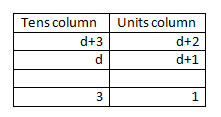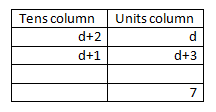#### You may also like### Consecutive Numbers

An investigation involving adding and subtracting sets of consecutive numbers. Lots to find out, lots to explore.### Calendar Capers

Choose any three by three square of dates on a calendar page...Make a set of numbers that use all the digits from 1 to 9, once and once only. Add them up. The result is divisible by 9. Add each of the digits in the new number. What is their sum? Now try some other possibilities for yourself!

# Making a Difference

##### Age 11 to 14Challenge Level

There were lots of good solutions to this question. Well done and thank you to everyone who submitted solutions! We couldn't include everyone's solutions, as there were too many, but well done to you all.

A number of you, including Fraser, Jacque, Dan and Jack, all from the Crypt School; Brendon and Ava, both from St Mary's Catholic High School; Lois, Jack, Oliver and Lewis from Kingsway Primary School; Hasan from Dixons Kings Academy and Sophie from Wallington High School for Girls found a number of the solutions, and many of them found all 12:

$\begin{eqnarray} 82-75 &=& 7\\ 85-72 &=& 13\\ 72-58 &=& 14\\ 82-57 &=& 25\\ 78-52 &=& 26\\ 57-28 &=& 29\\ 58-27 &=& 31\\ 87-52 &=& 35\\ 75-28 &=& 47\\ 78-25 &=& 53\\ 85-27 &=& 58\\ 87-25 &=& 62 \end{eqnarray}$

Lev, from the Assosciated Hebrew Schools Danilack, Canada, was able to explain how he started finding these systematically, by looking at all of those numbers where the tens digit of the top number is $8$, which gave him six subtractions.

Ella, from Maidstone Girls Grammar School, and Mr Dutton's Maths Group, from Hartley Primary Academy, both explained how they managed to find all twelve solutions in a systematic manner. Here is Ella's solution

In order to find the solution to this problem, I worked systematically. First of all, I worked out all the possible sums if your first digit of the bigger number was five, then I did the same with seven, then eight. I didn't try it with two because all the other numbers possible would be bigger than any possible with the number two and then you would get a negative number, which wouldn't work.

Seth, from the British International School of Boston, USA, had a different systematic method:

To solve the puzzle 'Making a Difference' I first thought about the numbers there were to subtract from. I arrived at the answer : 25, 27, 28, 57, 58, 52, 72, 75, 78, 82, 85, and 87. But, if you look at the number which is composed of just the two smallest numbers or the numbers which have 2 at the start, and check the combinations for the subtracted number, you will find that all combinations from that are negative. After that, you can just try all other combinations and you're done!

Jamie, from Shrewsbury International School in Thailand, used a different method to systematically find the solutions.

There are 4 numbers. In the first square, you are able to put 4 different numbers. Keep the 4. The second square can have 3 different numbers, since one number is already used. There are two numbers that can go into the third square. And the last square matches with the last number left. Multiplying $4 \times 3 \times 2 \times 1 = 24$ gives the number of orders. This is also called $4$ factorial, written as $4!$.

Zach was able to develop this to get to again show that there are 12 solutions:

$4!$ gives 24 possible 4-digit numbers. These then form the two 2-digit numbers, but since the answer must be positive, $28$ and $57$ gives the same sum as $57$ and $28$. This means that half the possible 24 combinations are duplicates, as you always have to take the smaller number from the bigger number to get a positive result.

Max and Ben, from St. Columba's Primary School, Australia both found that 3, 4, 5 and 6 work to give the stated set of numbers.

$\begin{eqnarray} 53-46 &=& 7\\ 63-54 = 45-36 &=& 9\\ 64-53 = 46-35 &=& 11\\ 56-43 &=& 13\\ 63-45 = 54-36 &=& 18\\ 65-43 = 56-34 &=& 22\\ 64-35 &=& 29\\ 65-34 &=& 31 \end{eqnarray}$

Jessica, from Wallington High School for Girls, was able to explain why any set of four consecutive numbers would give this set of answers.

The simplest idea I could think of to start with, was to use consecutive numbers. I realised that it didn't matter what consecutive numbers I used because the differences would cancel out. For example, using 1, 2, 3 and 4, 43 - 21 = 22. Using 5, 6, 7 and 8, 87 - 65 = 22. So when I tried 1, 2, 3, and 4, and crossed out all the negative answers, I got: 7, 9, 11, 13, 18, 22, 29 and 31.

Matty, Neo and Sienna, from the British School Manila, in the Philippines, Zach, Chris from Lakeside High School, USA, and Tomas from Wilson's School all used algebra to solve the final part. Here is a combination of their solutions:

For consecutive numbers where $a>b>c>d$:
$a = b+1$, $b = c+1$ and $c = d+1$.

Therefore $a=d+3$, $b=d+2$ and $c=d+1$.

For the largest gap, the tens digit of the top number must be as large as possible, and the tens digit must be as small as possible. This means the subtraction is $ab-cd$ (where $ab$ is the number with digits $a$ and $b$, rather than $a \times b$).The largest difference is therefore 31.

For the smallest gap it is $bd - ca$ because $a$ must be at the bottom because it is the biggest and you want to be taking away big numbers. It can't go in the bottom tens column because then the answer would be negative. b, the second biggest, can't accompany a because then it would also be a negative. c is the next biggest. This works out as positive.

Therefore the sum is:Note that there is a carry occurring here. The smallest difference is therefore 7.

Thank you again to everyone who submitted a solution, and well done!